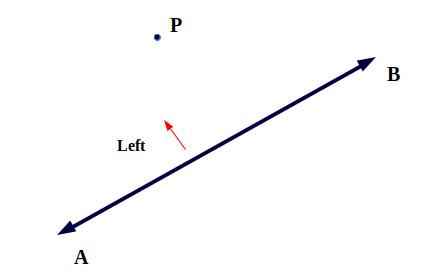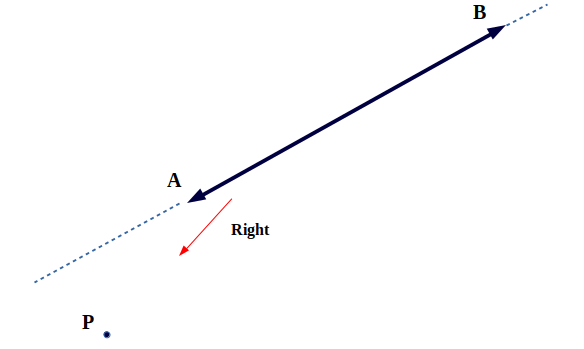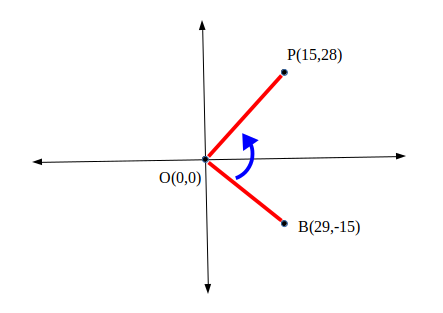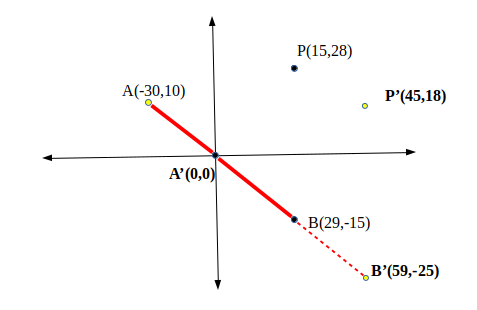# Direction of a Point from a Line Segment

Direction of given point P from a line segment simply means given the co-ordinates of a point P and line segment (say AB), and we have to determine the direction of point P from the line segment. That is whether the Point lies to the Right of Line Segment or to the Left of Line Segment.The point might lie behind the line segment, in that case we assume imaginary line by extending the line segment and determine the direction of point.* There are only three cases, either the point is on left side, or right side or on the line segment itself.
This is a very fundamental Problem and is commonly encountered for directions in online map
Example : Suppose a user A has to go to Point C in the following map, the user first reaches point B but after that how does user A know that whether he has to make a right turn or left turn.Knowing the direction of a point from a line segment also acts a building block to solve more complicated problem such as :

• Line segment Intersection : finding if two line segment intersect
• Convex Hull of a set of Points.

The co-ordinate system we’ll use is a cartesian plane, as most 2-Dimensional problem uses cartesian plane and since this is a 2-Dimensional Problem.
This Problem can be solved using cross-product of vector algebra
Cross-Product of two point A and B is : Ax * By – Ay * Bx
where Ax and Ay are x and y co-ordinates of A respectively. Similarly Bx and By are x and y co-ordinates of B respectively.
The Cross-Product has an interesting Property which will be used to determine direction of a point from a line segment. That is, the cross-product of two points is positive if and only if the angle of those point at origin (0, 0) is in counter-clockwise. And conversely the cross-product is negative if and only if the angle of those point at origin is in clockwise direction.
An example would certainly clarify it
In the following figure, the angle BOP is anti-clockwise and the cross-product of B X P = 29*28 – 15*(-15) = 1037 which is positive.This helps us to make a conclusion that a point on right side must a have positive cross-product and a point on left side must have a negative cross product. Also note that we assumed one point of line segment to be origin and hence we need to convert any three point system such that one point of line segment is origin.

Following example explains the concept
The three point A, B and P were converted into A’, B’ and P’ so as to make A as origin (this can be simply done by subtracting co-ordinates of A from point P and B), and then calculate the cross-product : 59*18 – (-25)*18 = 2187
Since this is positive, the Point P is on right side of line Segment AB.## C++

 `// C++ Program to Determine Direction of Point` `// from line segment` `#include ` `using` `namespace` `std;`   `// structure for point in cartesian plane.` `struct` `point {` `    ``int` `x, y;` `};`   `// constant integers for directions` `const` `int` `RIGHT = 1, LEFT = -1, ZERO = 0;`   `int` `directionOfPoint(point A, point B, point P)` `{` `    ``// subtracting co-ordinates of point A from` `    ``// B and P, to make A as origin` `    ``B.x -= A.x;` `    ``B.y -= A.y;` `    ``P.x -= A.x;` `    ``P.y -= A.y;`   `    ``// Determining cross Product` `    ``int` `cross_product = B.x * P.y - B.y * P.x;`   `    ``// return RIGHT if cross product is positive` `    ``if` `(cross_product > 0)` `        ``return` `RIGHT;`   `    ``// return LEFT if cross product is negative` `    ``if` `(cross_product < 0)` `        ``return` `LEFT;`   `    ``// return ZERO if cross product is zero. ` `    ``return` `ZERO; ` `}`   `// Driver code` `int` `main()` `{` `    ``point A, B, P;` `    ``A.x = -30;` `    ``A.y = 10; ``// A(-30, 10)` `    ``B.x = 29;` `    ``B.y = -15; ``// B(29, -15)` `    ``P.x = 15;` `    ``P.y = 28; ``// P(15, 28)`   `    ``int` `direction = directionOfPoint(A, B, P);` `    ``if` `(direction == 1)` `        ``cout << ``"Right Direction"` `<< endl;` `    ``else` `if` `(direction == -1)` `        ``cout << ``"Left Direction"` `<< endl;` `    ``else` `        ``cout << ``"Point is on the Line"` `<< endl;` `    ``return` `0;` `}`

## Java

 `// Java Program to Determine Direction of Point` `// from line segment` `class` `GFG ` `{`   `// structure for point in cartesian plane.` `static` `class` `point ` `{` `    ``int` `x, y;` `};`   `// constant integers for directions` `static` `int` `RIGHT = ``1``, LEFT = -``1``, ZERO = ``0``;`   `static` `int` `directionOfPoint(point A, ` `                            ``point B, point P)` `{` `    ``// subtracting co-ordinates of point A ` `    ``// from B and P, to make A as origin` `    ``B.x -= A.x;` `    ``B.y -= A.y;` `    ``P.x -= A.x;` `    ``P.y -= A.y;`   `    ``// Determining cross Product` `    ``int` `cross_product = B.x * P.y - B.y * P.x;`   `    ``// return RIGHT if cross product is positive` `    ``if` `(cross_product > ``0``)` `        ``return` `RIGHT;`   `    ``// return LEFT if cross product is negative` `    ``if` `(cross_product < ``0``)` `        ``return` `LEFT;`   `    ``// return ZERO if cross product is zero. ` `    ``return` `ZERO; ` `}`   `// Driver code` `public` `static` `void` `main(String[] args) ` `{` `    ``point A = ``new` `point(), ` `          ``B = ``new` `point(), P = ``new` `point();` `    ``A.x = -``30``;` `    ``A.y = ``10``; ``// A(-30, 10)` `    ``B.x = ``29``;` `    ``B.y = -``15``; ``// B(29, -15)` `    ``P.x = ``15``;` `    ``P.y = ``28``; ``// P(15, 28)`   `    ``int` `direction = directionOfPoint(A, B, P);` `    ``if` `(direction == ``1``)` `        ``System.out.println(``"Right Direction"``);` `    ``else` `if` `(direction == -``1``)` `        ``System.out.println(``"Left Direction"``);` `    ``else` `        ``System.out.println(``"Point is on the Line"``);` `    ``}` `}`   `// This code is contributed` `// by Princi Singh`

## Python3

 `# Python3 program to determine direction ` `# of point from line segment` ` `  `# Structure for point in cartesian plane.` `class` `point:` `    `  `    ``def` `__init__(``self``):` `        `  `        ``self``.x ``=` `0` `        ``self``.y ``=` `0` ` `  `# Constant integers for directions` `RIGHT ``=` `1` `LEFT ``=` `-``1` `ZERO ``=` `0` ` `  `def` `directionOfPoint(A, B, P):` `    `  `    ``global` `RIGHT, LEFT, ZERO` `    `  `    ``# Subtracting co-ordinates of ` `    ``# point A from B and P, to ` `    ``# make A as origin` `    ``B.x ``-``=` `A.x` `    ``B.y ``-``=` `A.y` `    ``P.x ``-``=` `A.x` `    ``P.y ``-``=` `A.y` ` `  `    ``# Determining cross Product` `    ``cross_product ``=` `B.x ``*` `P.y ``-` `B.y ``*` `P.x` ` `  `    ``# Return RIGHT if cross product is positive` `    ``if` `(cross_product > ``0``):` `        ``return` `RIGHT` `        `  `    ``# Return LEFT if cross product is negative` `    ``if` `(cross_product < ``0``):` `        ``return` `LEFT` ` `  `    ``# Return ZERO if cross product is zero` `    ``return` `ZERO`   `# Driver code ` `if` `__name__``=``=``"__main__"``:` `    `  `    ``A ``=` `point()` `    ``B ``=` `point()` `    ``P ``=` `point()` `    `  `    ``A.x ``=` `-``30` `    ``A.y ``=` `10` `# A(-30, 10)` `    ``B.x ``=` `29` `    ``B.y ``=` `-``15` `# B(29, -15)` `    ``P.x ``=` `15` `    ``P.y ``=` `28` `# P(15, 28)` ` `  `    ``direction ``=` `directionOfPoint(A, B, P)` `    `  `    ``if` `(direction ``=``=` `1``):` `        ``print``(``"Right Direction"``)` `    ``elif` `(direction ``=``=` `-``1``):` `        ``print``(``"Left Direction"``)` `    ``else``:` `        ``print``(``"Point is on the Line"``)`   `# This code is contributed by rutvik_56`

## C#

 `// C# Program to Determine Direction of Point` `// from line segment` `using` `System;` `using` `System.Collections.Generic;`   `class` `GFG ` `{`   `// structure for point in cartesian plane.` `public` `class` `point ` `{` `    ``public` `int` `x, y;` `};`   `// constant integers for directions` `static` `int` `RIGHT = 1, LEFT = -1, ZERO = 0;`   `static` `int` `directionOfPoint(point A, ` `                            ``point B, point P)` `{` `    ``// subtracting co-ordinates of point A ` `    ``// from B and P, to make A as origin` `    ``B.x -= A.x;` `    ``B.y -= A.y;` `    ``P.x -= A.x;` `    ``P.y -= A.y;`   `    ``// Determining cross Product` `    ``int` `cross_product = B.x * P.y - B.y * P.x;`   `    ``// return RIGHT if cross product is positive` `    ``if` `(cross_product > 0)` `        ``return` `RIGHT;`   `    ``// return LEFT if cross product is negative` `    ``if` `(cross_product < 0)` `        ``return` `LEFT;`   `    ``// return ZERO if cross product is zero. ` `    ``return` `ZERO; ` `}`   `// Driver code` `public` `static` `void` `Main(String[] args) ` `{` `    ``point A = ``new` `point(), ` `          ``B = ``new` `point(),` `          ``P = ``new` `point();` `    ``A.x = -30;` `    ``A.y = 10; ``// A(-30, 10)` `    ``B.x = 29;` `    ``B.y = -15; ``// B(29, -15)` `    ``P.x = 15;` `    ``P.y = 28; ``// P(15, 28)`   `    ``int` `direction = directionOfPoint(A, B, P);` `    ``if` `(direction == 1)` `        ``Console.WriteLine(``"Right Direction"``);` `    ``else` `if` `(direction == -1)` `        ``Console.WriteLine(``"Left Direction"``);` `    ``else` `        ``Console.WriteLine(``"Point is on the Line"``);` `    ``}` `}`   `// This code is contributed by 29AjayKumar`

Output:

```Right Direction

```

This article is contributed by Shubham Rana. If you like GeeksforGeeks and would like to contribute, you can also write an article using contribute.geeksforgeeks.org or mail your article to contribute@geeksforgeeks.org. See your article appearing on the GeeksforGeeks main page and help other Geeks.

Attention reader! Don’t stop learning now. Get hold of all the important DSA concepts with the DSA Self Paced Course at a student-friendly price and become industry ready.

My Personal Notes arrow_drop_up

Article Tags :
Practice Tags :

4

Please write to us at contribute@geeksforgeeks.org to report any issue with the above content.